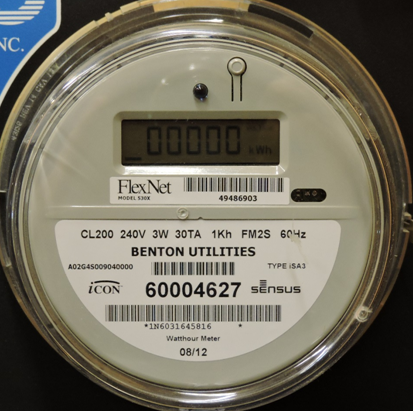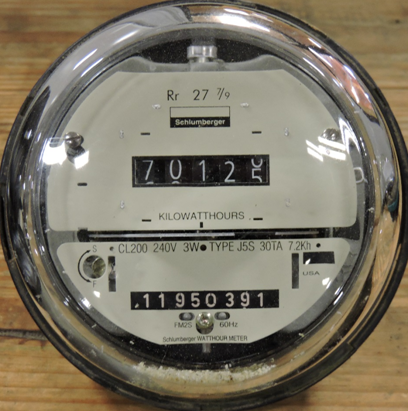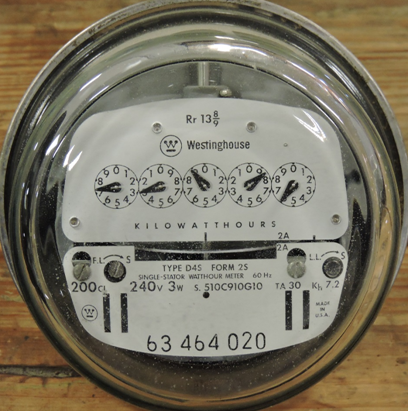Your electric meter is a precision instrument that measures the flow of electricity in units call kilowatt-hour (kWh). Electric meters are extremely accurate. Less than one-half of one percent of meters tested prove to be outside the acceptable error rate of plus or minus two percent. Your meter will be either digital or dial. Digital meters are read like the odometer of your car from left to right.

The correct reading for the digital meter below is 0.The correct reading for the odometer style meter below is 70125.To read a dial meter, read each dial separately from the right to the left and record the numbers in the same order, right to left. Start with the dial on the far right and record for each dial the number that the pointer has just passed.

The correct reading for the meter shown below would be 72886. When the dial is so near a number that it seems to point directly to it, look at the dial to the right. If the pointer on that dial has not passed zero, then you would record the lower number instead of the number the dial is near. If the pointer has passed zero, you would record the higher number.